# Order formulas for linear groups of degree two

This article gives a list of formulas for the orders of the general linear group of degree two and some other related groups, both for a finite field of size$q$ and for related rings.

For the related formulas for degrees other than two, as well as for more detailed explanations of the order formulas, see order formulas for linear groups.

## For a finite field of size$q$

### Formulas

In the formulas below, the field size is$q$. The characteristic of the field is a prime number$p$.$q$ is a prime power with underlying prime$p$. We let$r = \log_pq$, so$q = p^r$ and$r$ is a nonnegative integer.

Group Symbolic notation Order formula Order formula (maximally factorized) Order formula (expanded) Degree as polynomial in$q$ (same as algebraic dimension) Quick explanation for order
general linear group of degree two$GL(2,q)$ or$GL(2,\mathbb{F}_q)$$(q^2 - 1)(q^2 - q)$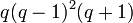$q(q-1)^2(q+1)$$q^4 - q^3 - q^2 + q$ 4 [SHOW MORE]
projective general linear group of degree two$PGL(2,q)$ or$PGL(2,\mathbb{F}_q)$$q(q^2 - 1)$$q(q-1)(q+1)$$q^3 - q$ 3 [SHOW MORE]
special linear group of degree two$SL(2,q)$ or$SL(2,\mathbb{F}_q)$$q(q^2 - 1)$$q(q-1)(q+1)$$q^3 - q$ 3 [SHOW MORE]
projective special linear group of degree two$PSL(2,q)$ or$PSL(2,\mathbb{F}_q)$$q(q^2 - 1)/\operatorname{gcd}(2,q-1)$$q(q^2 - 1)/2$ for$q$ odd$q(q^2 - 1)$ for$q$ even$q(q-1)(q+1)/\operatorname{gcd}(2,q-1)$$q(q-1)(q+1)/2$ for$q$ odd$q(q-1)(q+1)$ for$q$ even$(q^3 - q)/\operatorname{gcd}(2,q-1)$$(q^3 - q)/2$ for$q$ odd$q^3 - q$ for$q$ even
general semilinear group of degree two$\Gamma L(2,q)$ or$\Gamma L(2,\mathbb{F}_q)$$r(q^2 - 1)(q^2 - q)$$rq(q-1)^2(q+1)$$rq^4 - rq^3 -rq^2 + rq$ 4 [SHOW MORE]
projective semilinear group of degree two$P\Gamma L(2,q)$ or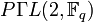$P\Gamma L(2,\mathbb{F}_q)$$rq(q^2 - 1)$$rq(q-1)(q+1)$$rq^3 - rq$ 3 [SHOW MORE]
general affine group of degree two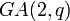$GA(2,q)$ or$AGL(2,q)$ or$GA(2,\mathbb{F}_q)$ or$AGL(2,\mathbb{F}_q)$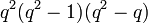$q^2(q^2 - 1)(q^2 - q)$$q^3(q-1)^2(q+1)$$q^6 - q^5 - q^4 + q^3$ 6 [SHOW MORE]
special affine group of degree two$SA(2,q)$ or$ASL(2,q)$ or$SA(2,\mathbb{F}_q)$ or$ASL(2,\mathbb{F}_q)$$q^2(q^3 - q)$$q^3(q - 1)(q + 1)$$q^5 - q^3$ 5 [SHOW MORE]

### Particular cases

The links are to the actual groups, which are not explicitly specified in order to save space.

Field size$q$ Field characteristic$p$$r$ so that$q = p^r$$|GL(2,q)|$$= (q^2 - 1)(q^2 - q)$$|PGL(2,q)|$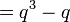$= q^3 - q$$|SL(2,q)|$$= q^3 - q$$|PSL(2,q)|$$= (q^3 - q)/\operatorname{gcd}(2,q-1)$$|\Gamma L(2,q)| =$$r(q^2 - 1)(q^2 - q)$$|P\Gamma L(2,q)| =$$r(q^3 - q)$$|GA(2,q)| =$$q^2(q^2 - 1)(q^2 - q)$
2 2 1 6 6 6 6 6 6 24
3 3 1 48 24 24 12 48 24 432
4 2 2 180 60 60 60 360 120 2880
5 5 1 480 120 120 60 480 120 12000
7 7 1 2016 336 336 168 2016 336 38304
8 2 3 3528 504 504 504 10584 1512 225792
9 3 2 5760 720 720 360 11520 1440 466560